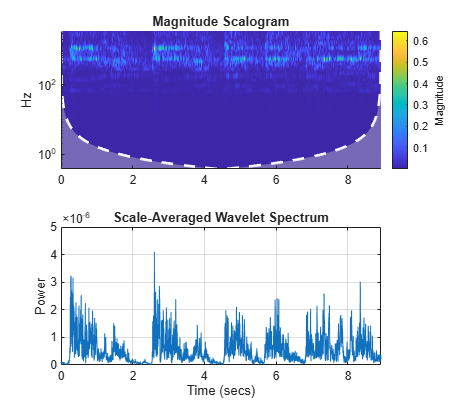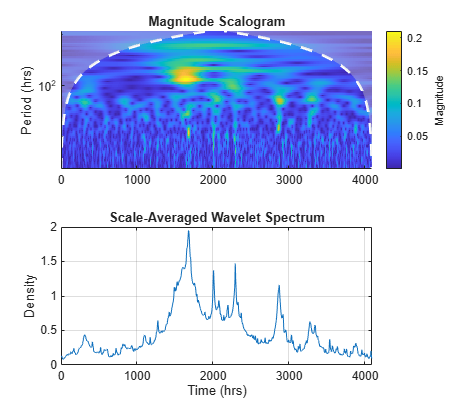# scaleSpectrum

Scale-averaged wavelet spectrum

## Syntax

``savgp = scaleSpectrum(fb,x)``
``savgp = scaleSpectrum(fb,cfs)``
``[savgp,scidx] = scaleSpectrum(___)``
``[___] = scaleSpectrum(___,Name,Value)``
``scaleSpectrum(___)``

## Description

example

````savgp = scaleSpectrum(fb,x)` returns the scale-averaged wavelet power spectrum of the signal `x` using the CWT filter bank `fb`. By default, `savgp` is obtained by scale-averaging the magnitude-squared scalogram over all scales.```
````savgp = scaleSpectrum(fb,cfs)` returns the scale-averaged wavelet spectrum for the CWT coefficients `cfs`. NoteWhen using this syntax, the power of the scale-averaged wavelet spectrum is normalized to equal the variance of the last signal processed by the filter bank object function `wt`. ```
````[savgp,scidx] = scaleSpectrum(___)` also returns the scale indices over which the scale-averaged wavelet spectrum is computed. If you do not specify `FrequencyLimits` or `PeriodLimits`, `scidx` is a vector from 1 to the number of scales. ```

example

````[___] = scaleSpectrum(___,Name,Value)` specifies additional options using name-value pair arguments. These arguments can be added to any of the previous input syntaxes. For example, `'Normalization','none'` specifies no normalization of the scale-averaged wavelet spectrum.```
````scaleSpectrum(___)` with no output arguments plots the scale-averaged wavelet power spectrum in the current figure.```

## Examples

collapse all

Load an audio file containing a fragment of Handel's "Hallelujah Chorus" sampled at 8192 Hz.

`load handel % To hear, type soundsc(y,Fs)`

Create a CWT filter bank that can be applied to the signal. Use the default Morse wavelet.

`fb = cwtfilterbank('SignalLength',length(y),'SamplingFrequency',Fs);`

Plot the scalogram and scale-averaged wavelet power spectrum using the default settings.

`scaleSpectrum(fb,y)`Load a time series of solar magnetic field magnitudes recorded hourly over the south pole of the sun by the Ulysses spacecraft from 21:00 UT on December 4, 1993 to 12:00 UT on May 24, 1994. See  pp. 218–220 for a complete description of this data. Create a CWT filter bank that can be applied to the data. Plot the scalogram and the scale-averaged wavelet spectrum.

```load solarMFmagnitudes fb = cwtfilterbank('SignalLength',length(sm),'SamplingPeriod',hours(1)); scaleSpectrum(fb,sm)```Obtain the scale-averaged wavelet spectrum of the signal using default values. By default, `scaleSpectrum` normalizes the power of the scale-averaged wavelet spectrum to equal the variance of the signal. Verify that the sum of the spectrum equals the variance of the signal.

```savg = scaleSpectrum(fb,sm); [var(sm) sum(savg)]```
```ans = 1×2 0.0448 0.0447 ```

Obtain the scale-averaged wavelet spectrum of the signal, but instead normalize the power as a probability density function. Verify that the sum is equal to 1.

```savg = scaleSpectrum(fb,sm,'Normalization','pdf'); sum(savg)```
```ans = 1.0000 ```

If you set `SpectrumType` to `'density'`, `scaleSpectrum` normalizes the weighted integral of the wavelet spectrum according to the value of `Normalization`. In this case, the spectrum mimics a probability density function whose integral, numerically evaluated, equals the value specified by `Normalization`.

Plot the scalogram and the scale-averaged wavelet spectrum with spectrum type `'density'` and `'pdf'` normalization.

```figure scaleSpectrum(fb,sm,'SpectrumType','density','Normalization','pdf')```To confirm the integral of the spectrum equals 1, first obtain the scale-averaged wavelet spectrum with `'density'` spectrum type and `'pdf'` normalization.

`savg = scaleSpectrum(fb,sm,'SpectrumType','density','Normalization','pdf');`

By default, the filter bank uses the analytic Morse (3,60) wavelet. Obtain the admissibility constant for the wavelet and numerically integrate the wavelet spectrum using the trapezoidal rule. Confirm that the integral equals 1.

```ga = 3; tbw = 60; be = tbw/ga; anorm = 2*exp(be/ga*(1+(log(ga)-log(be)))); cPsi = anorm^2/(2*ga).*(1/2)^(2*(be/ga)-1)*gamma(2*be/ga); numInt = 2/cPsi*1/length(sm)*trapz(1:length(savg),savg)```
```numInt = 1.0000 ```

## Input Arguments

collapse all

Continuous wavelet transform (CWT) filter bank, specified as a `cwtfilterbank` object.

Input data, specified as a real- or complex-valued vector. The input data `x` must have at least four samples.

Data Types: `single` | `double`
Complex Number Support: Yes

CWT coefficients, specified as a 2-D matrix or as an M-by-N-by-2 array. `cfs` should be the output of the `wt` object function of the CWT filter bank `fb`.

Data Types: `single` | `double`
Complex Number Support: Yes

### Name-Value Arguments

Specify optional pairs of arguments as `Name1=Value1,...,NameN=ValueN`, where `Name` is the argument name and `Value` is the corresponding value. Name-value arguments must appear after other arguments, but the order of the pairs does not matter.

Before R2021a, use commas to separate each name and value, and enclose `Name` in quotes.

Example: `scaleSpectrum(fb,x,'FrequencyLimits',[0.2 0.4])` returns the scale-averaged wavelet spectrum averaged over the frequency limits ```[0.2 0.4]```.

Normalization of the scale-averaged wavelet spectrum, specified as a comma-separated pair consisting of `'Normalization'` and one of the following:

• `'var'` — Normalize to equal the variance of the time series `x`. If you provide the `cfs` input, the `scaleSpectrum` function uses the variance of the last time series processed by the filter bank object function `wt`.

• `'pdf'` — Normalize to equal 1.

• `'none'` — No normalization is applied.

Type of wavelet spectrum to return, specified as a comma-separated pair consisting of `'SpectrumType'` and either `'power'` or `'density'`. If specified as `'power'`, the averaged sum of the scale-averaged wavelet spectrum over all scales is normalized according to the value specified in '`Normalization`'. If specified as `'density'`, the weighted integral of the wavelet spectrum over all scales is normalized according to the value specified in '`Normalization`'.

Frequency limits over which the magnitude-squared scalogram is averaged, specified as a comma-separated pair consisting of `'FrequencyLimits'` and a two-element vector with nondecreasing elements. The `FrequencyLimits` values must lie between the lowest and highest center frequencies returned by the `centerFrequencies` object function of `fb`. The base 2 logarithm of the ratio of the maximum frequency to the minimum frequency must be greater than or equal to 1/NV, where NV is the value of the '`VoicesPerOctave`' property of the filter bank `fb`.

If a region of the specified limits falls outside the frequency limits of the filter bank `fb`, `scaleSpectrum` truncates computations to within the range specified by `centerFrequencies(fb)`. `FrequencyLimits` cannot be completely outside of the Nyquist range.

Period limits over which the magnitude-squared scalogram is averaged, specified as a comma-separated pair consisting of `'PeriodsLimits'` and a two-element vector with nondecreasing durations. The elements of `PeriodLimits` agree in type and format with the '`SamplingPeriod`' property of the filter bank `fb`. The `SamplingPeriod` values must lie between the lowest and highest center periods returned by the `centerPeriods` object function of `fb`. The base 2 logarithm of the ratio of the minimum period to the maximum period must be less than or equal to -1/NV, where NV is the value of the '`VoicesPerOctave`' property of the filter bank `fb`.

If a region of the specified limits falls outside the period limits of the filter bank `fb`, `scaleSpectrum` truncates computations to within the range specified by `centerPeriods(fb)`. `SamplingPeriod` cannot be completely outside the Nyquist range of [2*Ts,N*Ts], where `Ts` is the '`SamplingPeriod`' and N is the signal length.

## Output Arguments

collapse all

Scale-averaged wavelet power spectrum, returned as a real-valued vector or real-valued 3-D array. If `x` is real-valued, `savgp` is a 1-by-N vector where N is the length of `x`. If `x` is complex-valued, `savgp` is a 1-by-N-by-2 array, where the first page is the scale-averaged wavelet spectrum for the positive scales (analytic part or counterclockwise component), and the second page is the scale-averaged wavelet spectrum for the negative scales (anti-analytic part or clockwise component).

Scale indices over which the scale-average wavelet spectrum is computed, returned as a vector. If you do not specify '`FrequencyLimits`' or '`PeriodLimits`', `scidx` is a vector from 1 to the number of scales.

 Torrence, Christopher, and Gilbert P. Compo. “A Practical Guide to Wavelet Analysis.” Bulletin of the American Meteorological Society 79, no. 1 (January 1, 1998): 61–78. https://doi.org/10.1175/1520-0477(1998)079<0061:APGTWA>2.0.CO;2.

 Percival, Donald B., and Andrew T. Walden. Wavelet Methods for Time Series Analysis. Cambridge Series in Statistical and Probabilistic Mathematics. Cambridge ; New York: Cambridge University Press, 2000.

 Lilly, J.M., and S.C. Olhede. “Higher-Order Properties of Analytic Wavelets.” IEEE Transactions on Signal Processing 57, no. 1 (January 2009): 146–60. https://doi.org/10.1109/TSP.2008.2007607.